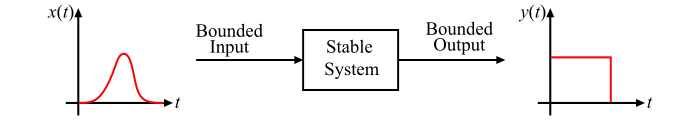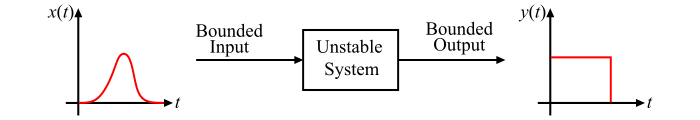# Signals and Systems: Stable and Unstable System

## Stable System or BIBO Stable System

A system is called a BIBO (bounded input bounded output) stable system or simply stable system, if and only if every bounded input produces a bounded output. The output of a stable system does not change unreasonably.The stability of a system indicates the usefulness of the system. The stability of a system can be determined from the impulse response of the system. The impulse response of the system is nothing but the output of the system for a unit impulse input.

If the impulse response of the system is absolutely integrable for a continuoustime system or absolutely summable for a discrete time system, then the system is a stable system.

Let an input signal x(t) is bounded signal, i.e.,

|𝑥(𝑡)| < 𝐾𝑥 < ∞ for − ∞ < 𝑡 < ∞

Where, 𝐾𝑥 is a positive real number. Then, if

|𝑦(𝑡)| < 𝐾𝑦 < ∞

That is, the output of the system y(t) is also bounded, then the system is called BIBO stable system.

## Unstable System

If a system does not satisfy the BIBO stability condition, the system is called the unstable system. Therefore, for a bounded input, it is not necessary that the unstable system produces a bounded output. Thus, we can say that a system is unstable even if one bounded input generates an unbounded output.## Solved Example

Find whether the given systems are stable or unstable −

• 𝑦(𝑡) = 𝑒𝑥(𝑡) for |𝑥(𝑡)| ≤ 6

• $\mathrm{h(t)=\frac{1}{RC}e^{\frac{-t}{RC}}u(t)}$

• 𝑦(𝑡) = (𝑡 + 7)𝑢(𝑡)

• ℎ(𝑡) = 𝑒3𝑡𝑢(𝑡)

## Solution

• The output of the given system is,

𝑦(𝑡) = 𝑒𝑥(𝑡) for |𝑥(𝑡)| ≤ 6

The input of the given system is bounded, i.e.,

|𝑥(𝑡)| ≤ 6

Therefore, to the system be stable, the output must be bounded.

For the given system, the output y(t) becomes,

𝑒−6 ≤ 𝑦(𝑡) ≤ 𝑒6

Thus, the output y(t) is also bounded. Hence the system is stable.

• The given system is

$$\mathrm{h(t)=\frac{1}{RC}e^{\frac{-t}{RC}}u(t)}$$

For stability of the system,

$$\mathrm{\int_{-\infty }^{\infty}\: \left | h(t) \right |dt< \infty }$$

For the given system,

$$\mathrm{\int_{-\infty }^{\infty}\: \left | h(t) \right |dt=\int_{-\infty }^{\infty}\:\left | \frac{1}{RC}e^{\frac{-t}{RC}}u(t) \right |dt=\int_{0}^{\infty}\:\left | \frac{1}{RC}e^{\frac{-t}{RC}} \right |dt }=1< \infty$$

Therefore, the given system is a stable system.

• The given system is

𝑦(𝑡) = (𝑡 + 7)𝑢(𝑡)

⟹ 𝑦(𝑡) = (𝑡 + 7); 𝑡 ≥ 0

Hence,

for 𝑡 → ∞; 𝑦(𝑡) → ∞

Thus, the output of the system increases without any bound. Therefore, the given system is an unstable system.

• The output of the given system is

ℎ(𝑡) = 𝑒3𝑡𝑢(𝑡)

For stability of the system,

$$\mathrm{\int_{-\infty }^{\infty}\: \left | h(t) \right |dt=\int_{-\infty }^{\infty}\left | e^{3t}u(t) \right |dt=\int_{0 }^{\infty}\left | e^{3t} \right |dt}$$ $$\mathrm{\Rightarrow \int_{-\infty }^{\infty}\: \left | h(t) \right |dt=\left [ \frac{e^{3t}}{3} \right ]_{0}^{\infty }=\left [ \frac{e^{\infty }}{3}- \frac{e^{0}}{3}\right ]=\infty }$$

The impulse response of the given system is not absolutely integrable. Therefore, the given system is an unstable system.